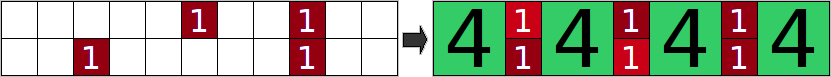"4Blocks" is a two player cooperative game played on a special board. The board is a grid composed of 1x1 square cells. There are two different kinds of blocks: '1' blocks and '4' blocks. '1' blocks are 1x1, and '4' blocks are 2x2:You must place blocks on the board so that their sides are aligned to the grid lines and no two blocks overlap. The final score is the sum of the values in each cell. '1' blocks are worth 1 point, and '4' blocks are worth 16 points because they cover 4 cells and each cell is worth 4 points.Your friend has taken his turn and placed a number of '1' blocks on the board. The current configuration is given in the vector grid. The j-th character of the i-th element of grid is '.' if the cell at row i, column j is empty, and '1' if your friend placed a '1' block in that cell. It is now your turn, and you can place any number of '1' or '4' blocks on the board, but you cannot remove any of the blocks that have already been placed. Return the maximum score that can be achieved. For example, the following images show one possible starting state, and the optimal placement of blocks from that state:The final score would be 4*16 + 6*1 = 70想法：求解最大的得分，也就是求出在这些空格中，可以摆放的最大的blocks的个数c，然后 16*c + n*m - 4*c既是其最大的得分。采用动态规划求解，设dp[x][y][s]表示在从方格(x,y)开始，y列的前一列的摆放状态为s,按列遍历到最后，能够摆放blocks最多有多少个。转移方程：(x,y)的摆放状态只与前一列相关。如果（x,y）被blocks覆盖，即判断前一列状态s&(1< g;    int dp(int x,int y,int mask)    {        int &ret = a[x][y][mask];        if(ret >= 0) return ret;        ret = 0;        if(y == m-1) return ret = 0;        if(x == n) return ret = dp(0,y+1,mask);        int nm = mask;        if(mask & (1 << x))        {            nm ^= (1< ret)            ret = temp;        return ret;    }    int maxScore(vector grid)    {        g = grid;        n = (int)g.size();        m = (int)g.size();        memset(a,0xff,sizeof(a));                int c = dp(0,0,0);        printf("%d\n",c);        return 12 * c + n*m;    }};
posted on 2009-07-09 16:00 newstar 阅读(68) 评论(0)  编辑 收藏 引用